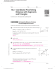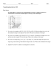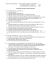HSM12CC_GM_06_09_CM

Transcription

HSM12CC_GM_06_09_CM
6-9
Proofs Using
Coordinate Geometry
Vocabulary
Review
1. Circle the Midpoint Formula for a segment in the coordinate plane. Underline the
Distance Formula for a segment in the coordinate plane.
M5 ¢
x1 1 x2 y1 1 y2
,
≤
2
2
d 5 "(x2 2 x1)2 1 (y2 2 y1)2
y 2y
m 5 x2 2 x1
2
1
2. Circle the Midpoint Formula for a segment on a number line. Underline the
Distance Formula for a segment on a number line.
M5
x1 1 x2
2
d 5 |x1 2 x2|
m5
x1 2 x2
2
Vocabulary Builder
VEHR
x and y are
often used as
variables.
ee uh bul
Related Words: vary (verb), variable (adjective)
Definition: A variable is a symbol (usually a letter) that represents one
or more numbers.
Math Usage: A variable represents an unknown number in equations
and inequalities.
Underline the correct word to complete each sentence.
3. An interest rate that can change is a variable / vary interest rate.
4. You can variable / vary your appearance by changing your hair color.
5. The amount of daylight variables / varies from summer to winter.
6. Circle the variable(s) in each expression below.
3n
41x
p2 2 2p
4
y
7. Cross out the expressions that do NOT contain a variable.
21m
Chapter 6
36 4 (2 ? 3)
9a2 2 4a
178
8 2 (15 4 3)
variable (noun)
Problem 1 Writing a Coordinate Proof
Got It? Reasoning You want to prove that the midpoint of the hypotenuse of a
right triangle is equidistant from the three vertices. What is the advantage of using
coordinates O(0, 0), E(0, 2b), and F(2a, 0) rather than O(0, 0), E(0, b), and F(a, 0)?
8. Label each triangle.
y
E(0,
y
)
, 2b)
E(
M
M
x
O (0, 0)
F(a,
x
O (0, 0)
)
9. Use the Midpoint Formula M 5 ¢
M in each triangle.
x1 1 x2 y1 1 y2
,
≤ to find the coordinates of
2
2
Fisrt Triangle
a
a10
2 ,
, 0)
F(
Second Triangle
1
2
a
b 5 a 2,
2
b
a
1
,
2
0 1 2b
2 b 5(
, b)
10. Use the Distance Formula, d 5 "(x2 2 x1)2 1 (y2 2 y1)2 and your answers to
Exercise 9 to verify that EM 5 FM 5 OM for the first triangle.
EM
FM
OM
11. Use the Distance Formula, d 5 "(x2 2 x1)2 1 (y2 2 y1)2 and your answers to
Exercise 9 to verify that EM 5 FM 5 OM for the second triangle.
EM
FM
OM
12. Which set of coordinates is easier to use? Explain.
_______________________________________________________________________
_______________________________________________________________________
179
Lesson 6-9
Writing a Coordinate Proof
Problem 2
Got It? Write a coordinate proof of the Triangle
y
Midsegment Theorem (Theorem 5-1).
B(
,
)
Given: E is the midpoint of AB and
F is the midpoint of BC
,
F(
Prove: EF 6 AC, EF 5 12AC
Use the diagram at the right.
)
+
E(
,
)
x
13. Label the coordinates of point C.
O C(
,
)
,
)
b 5(
,
A(
14. Reasoning Why should you make the coordinates
of A and B multiples of 2?
_______________________________________________________________________
_______________________________________________________________________
15. Label the coordinates of A and B in the diagram.
16. Use the Midpoint Formula to find the coordinates of E and F. Label the
coordinates in the diagram.
a
coordinates of F
+
+
,
2
b 5(
2
,
)
a
+
2
+
,
2
17. Use the Slope Formula to determine whether EF 6 AC.
2
slope of EF 5
5
2
2
slope of AC 5
5
2
18. Is EF 6 AC? Explain.
_______________________________________________________________________
_______________________________________________________________________
1
19. Use the Distance Formula to determine whether EF 5 2 AC.
EF 5
Å
AC 5
Å
(
20. 12 AC 5 12 ?
Chapter 6
2
(
2
)2 1 (
)2 1 (
5
2
2
)2 5 Î (a)2 1 (0)2 5
)2 5 Î (2a)2 1 (0)2 5
5 EF
180
)
coordinates of E
Lesson Check • Do you know HOW?
Use coordinate geometry to prove that the diagonals of a rectangle are congruent.
y
21. Draw rectangle PQRS with P at (0, 0).
22. Label Q(a,
), R(
, b), and S(
,
).
23. Complete the Given and Prove statements.
Given: PQRS is a
x
Prove: PR >
.
24. Use the Distance Formula to find the length of eatch diagonal.
PR 5
QS 5
Ä
(
2
)2 1 (
2
)2 5
Ä
(
2
)2 1 (
2
)2 5
25. PR 5
, so PR >
.
Lesson Check • Do you UNDERSTAND?
y
Error Analysis Your classmate places a trapezoid on the coordinate
plane. What is the error?
P(b, c) Q(a − b, c)
26. Check whether the coordinates are for an isosceles trapezoid.
OP 5
QR 5
Ä
Ä
(b 2
)2 1 (c 2
(a 2
x
O
)2 5
)2 1 (0 2
R(a, 0)
)2 5
27. Does the trapezoid look like an isosceles triangle?
Yes / No
_______________________________________________________________________
Math Success
Check off the vocabulary words that you understand.
proof
theorem
coordinate plane
coordinate geometry
Rate how well you can prove theorems using coordinate geometry.
Need to
review
0
2
4
6
8
Now I
get it!
10
181
Lesson 6-9

Page 1 Proving a triangle is a right triangle Method 1: Show two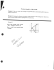Coordinate Geometry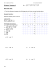Algebra with shapes How to . . .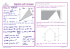Reteach Sec 1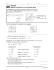5.1 Midsegment Theorem and Coordinate Proof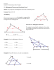Solve the problems below to answer the riddle: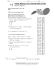readme - Computer Vision Lab Dresden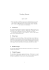HSM12CC_GM_06_08_CM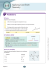Time: 3 to 3 ½ hours M.M.:90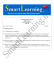See details. - Math Awareness Month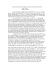The Midpoint Formula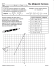Geometry Worksheet Name: Hodge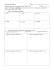STUDY GUIDE for Unit 1 Test Geometry 1. Z?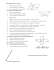10.3 Coordinate Proof Using Distance with Segments and Triangles